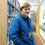Complex roots part 3 - Product of the roots

$\prod_{x = 0}^{n - 1}{Z_x} =~ ?$

The last note proved that the sum of all the roots is equal to zero. This note is going to explore what answer the product of all the roots gives.

Let's start.

$Z_x = \sqrt[n]{z} = \sqrt[2n]{a^2 + b^2} \cdot \text{cis}\left(\frac{\arctan \frac{b}{a}}{n} + \frac{2x\pi}{n}\right)$

$\prod_{x = 0}^{n - 1}{Z_x} = Z_0Z_1Z_2\cdots Z_{n - 1}$

There are $n$ terms.

$\prod_{x = 0}^{n - 1}{Z_x} = \sqrt[2n]{a^2 + b^2}^n \cdot \text{cis}\left(\frac{\arctan\frac{b}{a}}{n}\right)\text{cis}\left(\frac{\arctan\frac{b}{a}}{n} + \frac{2\pi}{n}\right)\text{cis}\left(\frac{\arctan\frac{b}{a}}{n} + \frac{4\pi}{n}\right)\cdots \text{cis}\left(\frac{\arctan\frac{b}{a}}{n} + \frac{2(n - 1)\pi}{n}\right)$

$\prod_{x = 0}^{n - 1}{Z_x} = \sqrt{a^2 + b^2} \cdot \text{cis}\left(n\frac{\arctan\frac{b}{a}}{n} + \frac{2\pi + 4\pi + \cdots + 2(n - 1)\pi}{n}\right)$

$2\pi + 4\pi + \cdots + 2(n - 1)\pi = 0 + 2\pi + 4\pi + \cdots + 2(n - 1)\pi$

There is now $n$ terms rather than $n - 1$ terms.

$\sum_{x = 0}^{n - 1}{2x\pi} = \frac{n(2\pi)(n - 1)}{2}$

$\sum_{x = 0}^{n - 1}{2x\pi} = n\pi(n - 1)$

$\sum_{x = 0}^{n - 1}{2x\pi} = n^2\pi - n\pi$

$\prod_{x = 0}^{n - 1}{Z_x} = \sqrt{a^2 + b^2} \cdot \text{cis}\left(\arctan\frac{b}{a} + \frac{n^2\pi - n\pi}{n}\right)$

$\prod_{x = 0}^{n - 1}{Z_x} = \sqrt{a^2 + b^2} \cdot \text{cis}\left(\arctan\frac{b}{a} + n\pi - \pi\right)$

$\prod_{x = 0}^{n - 1}{Z_x} = \sqrt{a^2 + b^2} \cdot \text{cis}\left(\arctan\frac{b}{a}\right)\text{cis}(n\pi - \pi)$

$\sqrt{a^2 + b^2} \cdot \text{cis}\left(\arctan \frac{b}{a}\right) = re^{\theta i} = z$

$\prod_{x = 0}^{n - 1}{Z_x} = z\text{cis}(n\pi - \pi)$

$\prod_{x = 0}^{n - 1}{Z_x} = z\frac{\text{cis}(n\pi)}{\text{cis}(\pi)}$

$\text{cis}(\pi) = \cos(\pi) + i\sin(\pi) = -1 + 0i = -1$

$\prod_{x = 0}^{n - 1}{Z_x} = z\frac{\text{cis}(n\pi)}{-1}$

$\prod_{x = 0}^{n - 1}{Z_x} = -z\text{cis}(n\pi)$

$\prod_{x = 0}^{n - 1}{Z_x} = -z\text{cis}(\pi)^n$

$\prod_{x = 0}^{n - 1}{Z_x} = -z(-1)^n$

$\prod_{x = 0}^{n - 1}{Z_x} = -(-1)^nz$

$\prod_{x = 0}^{n - 1}{Z_x} = (-1)^{n + 1}z$

In conclusion, if $n$ is even then the product of the roots is $-z$, if $n$ is odd then the product of the roots is $z$.

$\text{When }n\text{ is an even number}:~\prod_{x = 0}^{n - 1}{Z_x} = -z$

$\text{When }n\text{ is an odd number}:~\prod_{x = 0}^{n - 1}{Z_x} = z$

Hope you enjoyed the noteNote by Jack Rawlin
3 years, 7 months ago

This discussion board is a place to discuss our Daily Challenges and the math and science related to those challenges. Explanations are more than just a solution — they should explain the steps and thinking strategies that you used to obtain the solution. Comments should further the discussion of math and science.

When posting on Brilliant:

• Use the emojis to react to an explanation, whether you're congratulating a job well done , or just really confused .
• Ask specific questions about the challenge or the steps in somebody's explanation. Well-posed questions can add a lot to the discussion, but posting "I don't understand!" doesn't help anyone.
• Try to contribute something new to the discussion, whether it is an extension, generalization or other idea related to the challenge.
• Stay on topic — we're all here to learn more about math and science, not to hear about your favorite get-rich-quick scheme or current world events.

MarkdownAppears as
*italics* or _italics_ italics
**bold** or __bold__ bold

- bulleted
- list

• bulleted
• list

1. numbered
2. list

1. numbered
2. list
Note: you must add a full line of space before and after lists for them to show up correctly
paragraph 1

paragraph 2

paragraph 1

paragraph 2

> This is a quote
This is a quote
# I indented these lines
# 4 spaces, and now they show
# up as a code block.

print "hello world"
# I indented these lines
# 4 spaces, and now they show
# up as a code block.

print "hello world"
MathAppears as
Remember to wrap math in $$...$$ or $...$ to ensure proper formatting.
2 \times 3 $2 \times 3$
2^{34} $2^{34}$
a_{i-1} $a_{i-1}$
\frac{2}{3} $\frac{2}{3}$
\sqrt{2} $\sqrt{2}$
\sum_{i=1}^3 $\sum_{i=1}^3$
\sin \theta $\sin \theta$
\boxed{123} $\boxed{123}$

Sort by: